# Measure and classify an angle

A line that has one defined endpoint is called a ray and extends endlessly in one direction. A ray is named after the endpoint and another point on the ray e.g.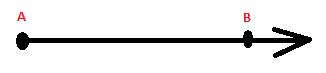$\overset{\rightarrow }{AB}$

The angle that is formed between two rays with the same endpoint is measured in degrees. The point is called the vertex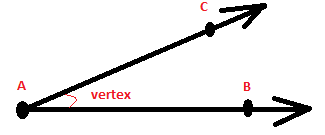The vertex is written as

$\measuredangle CAB$

In algebra we used the coordinate plane to graph and solve equations.  You can plot lines, line segments, rays and angles in a coordinate plane.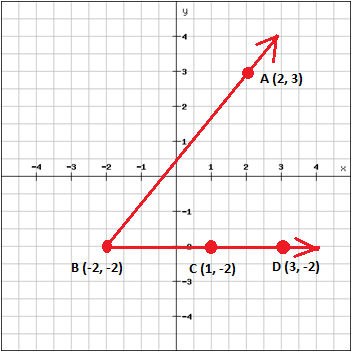In the coordinate plane above we have two rays

$\overset{\rightarrow }{BA}\: \: and\: \: \overset{\rightarrow }{BD}$

That form an angle with the vertex in point B.

You can use the coordinate plane to measure the length of a line segment. Point B is at (-2, -2) and C (1. -2). The distance between the two points is 1 - (-2) = 3 units.

Angles can be either straight, right, acute or obtuse.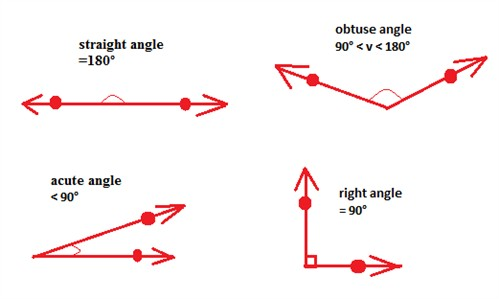An angle is a fraction of a circle where the whole circle is 360°. A straight angle is the same as half the circle and is 180° whereas a right angle is a quarter of a circle and is 90°.

You measure the size of an angle with a protractor.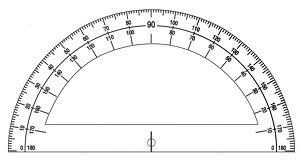Two angles with the same measure are called congruent angles. Congruent angles are denoted as

$\angle A\cong \angle B$

Or could be shown by an arc on the figure to indicate which angles that are congruent.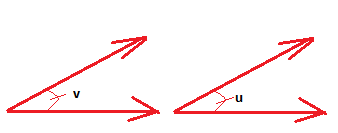Two angles whose measures together are 180° are called supplementary e.g. two right angles are supplementary since 90° + 90° = 180°.

Two angles whose measures together are 90° are called complementary.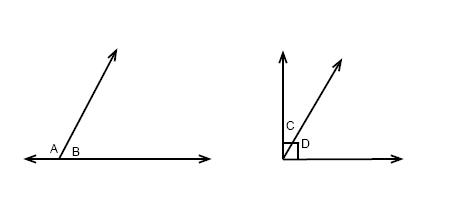$m\angle A+m\angle B=180^{\circ}$

$m\angle C+m\angle D=90^{\circ}$

## Video lesson

Measure the size of the angle